Leverage Point and Outlier Detection

The QUANTREG procedure uses robust multivariate location and scale estimates for leverage-point detection.

Mahalanobis distance is defined as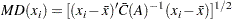where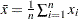and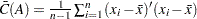are the empirical multivariate location and scale. Here,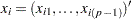does not include the intercept variable. The relationship between the Mahalanobis distanceand the matrix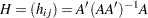is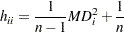Robust distance is defined as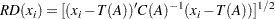where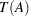and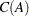are robust multivariate location and scale estimates computed with the minimum covariance determinant (MCD) method of Rousseeuw and Van Driessen (1999).

These distances are used to detect leverage points. You can use the DIAGNOSTICS and LEVERAGE options in the MODEL statement to request leverage-point and outlier diagnostics. Two new variables, Leverage and Outlier, are created and saved in an output data set specified in the OUTPUT statement.

Let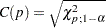be the cutoff value. The variable LEVERAGE is defined as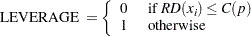You can specify a cutoff value with the LEVERAGE option in the MODEL statement.

Residuals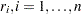, based on quantile regression estimates are used to detect vertical outliers. The variable OUTLIER is defined as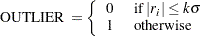You can specify the multiplier k of the cutoff value with the CUTOFF= option in the MODEL statement. You can specify the scale
with the SCALE= option in the MODEL statement. By default,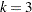and the scaleis computed as the corrected median of the absolute residuals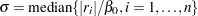, where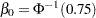is an adjustment constant for consistency with the normal distribution.

An ODS table called DIAGNOSTICS contains these two variables.